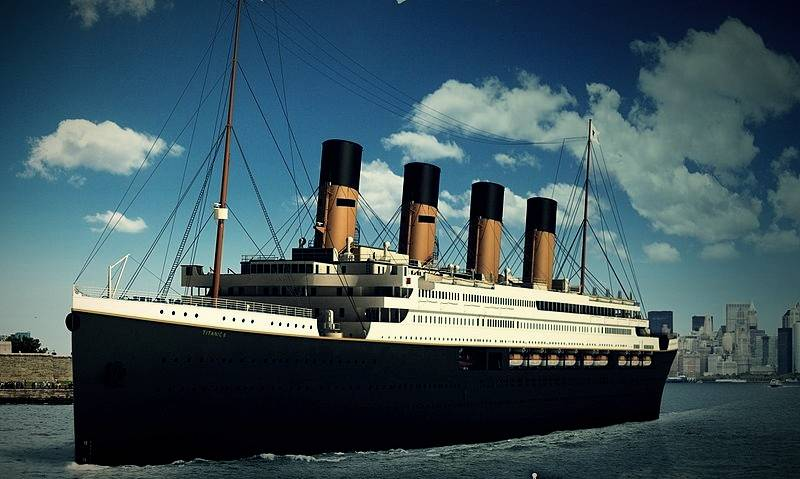December 6, 2023

# Brilliant is no longer! Oscars ceremony 2020 hits record lowAlthough the Korean film “Parasite” won the 92nd Academy Awards, Korean netizens are really happy, but still cannot hide an embarrassing fact.

In 2014, 43.74 million viewers brought the ratings for the Oscars to a small peak in ten years. However, it has fallen for 4 consecutive years, and in 2018 it has dropped to a record low of 26.5 million. In 2019, for some reason, there was no host for the Oscars, and the number of viewers rose by 10%.

Although, as in 2019, there is no host this year, the ratings have dropped by 20%, a record low, and 23.6 million viewers are much lower than in 2018.In 1997, “Titanic” was nominated for 14 Oscars, and finally won the 70th Oscar for best film, best director, best photography and other 11 awards. The Oscars ceremony that year saw 55.24 million visitors, the highest in history.

### Here are the audiences of the previous Oscars since 1974.

Number of viewers in the year
2020  =  23.6 million
2019  =  29.6 million
2018  =  26.5 million
2017   =  32.9 million
2016    = 34.3 million
2015  = 37.26 million
2014 =  44.74 million 2013 407.6 million
2012  = 39.46 million
2011  = 377.919 million
2010  = 416.9 million
2009 =  36.31 million 2008 3200.6 million
2007  = 40.172 million
2006 = 38.936 million
2005 = 4213.9 Wan

### Oscars ceremony 2020: 2004  = 43531000 “Lord of the Rings” 11 nominations for

2003  = 33,043,000
2002  = 41,782,000
2001  = 42,944,000
2000  = 46,333,000
1999  = 45,615,000
1998  = 55,249,000 “Titanic” 14 nominations for
1997  = 40,075,000
1996  = 44,867,000
1995  = 48,279,000
1994  = 45,083,000
1993  = 45.735 million
1992  = 44.406 million
1991 = 4272.7 million
1990  = 40.375 million
1989  = 42.619 million
1988  = 42.227 million
1987  = 37.19 million
1986  = 377.757 million
1985  = 38.855 million
1984  = 42.205 million
1983  = 53.235 million ET. “ Alien ” 9 nominations
1982  = 46.245 million
1981  = 3991.9 million
1980  = 4897.8 million
1979  = 4630.1 million
1978  = 4850.1 million
1977  = 39.719 million
1976  = 46.751 million
1975  = 48.127 million
1974  = 447.12,000

READ ALSO:   Hyundai Motor presents an updated car Veloster N